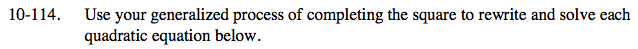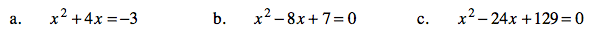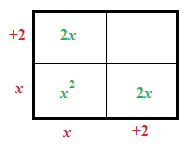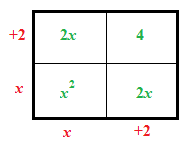### Home > AC > Chapter 10 > Lesson 10.3.2 > Problem10-114

10-114.Create a generic rectangle.What number needs to be added to both sides to complete the square?(x + 2)² = −3 + 4

(x + 2)² = 1

x = −3 or −1

Subtract 7 from both sides.

Follow the steps in part a.

(x − 4)² = 9

x = 1 or 7

Follow the steps in part (b).